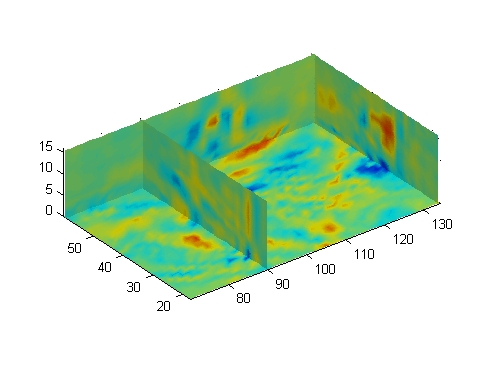MATLAB Function Referencedivergence

Computes the divergence of a vector field

Syntax

• ```div = divergence(X,Y,Z,U,V,W)
div = divergence(U,V,W)
div = divergence(X,Y,U,V)
div = divergence(U,V)
```

Description

```div = divergence(X,Y,Z,U,V,W) ``` computes the divergence of a 3-D vector field `U`, `V`, `W`. The arrays `X`, `Y`, `Z` define the coordinates for `U`, `V`, `W` and must be monotonic and 3-D plaid (as if produced by `meshgrid`).

```div = divergence(U,V,W) ``` assumes `X`, `Y`, and `Z` are determined by the expression

• ```[X Y Z] = meshgrid(1:n,1:m,1:p)
```

where `[m,n,p] = size(U)`.

```div = divergence(X,Y,U,V) ``` computes the divergence of a 2-D vector field `U`, `V`. The arrays `X`, `Y` define the coordinates for `U`, `V` and must be monotonic and 2-D plaid (as if produced by `meshgrid`).

```div = divergence(U,V) ``` assumes `X` and `Y` are determined by the expression

• ```[X Y] = meshgrid(1:n,1:m)
```

where `[m,n] = size(U)`.

Examples

This example displays the divergence of vector volume data as slice planes using color to indicate divergence.

• ```load wind
div = divergence(x,y,z,u,v,w);
slice(x,y,z,div,[90 134],,);
shading interp
daspect([1 1 1])
camlight```

See Also

`streamtube`, `curl`, `isosurface`

Volume Visualization for related functions

Displaying Divergence with Stream Tubes for another exampledisp (timer) dlmread© 1994-2005 The MathWorks, Inc.